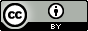## Unfortunate List

```-----BEGIN PGP SIGNED MESSAGE-----
Hash: SHA512

```
by Javantea
July 28, 2015

Update July 29, 2015
Javantea
@Javantea

My research into polynomials has led to a 3x3 captcha solver today. It's very fast. My next attempt will be a 6x6 captcha solver.

4:39 PM - 29 Jul 2015

Rather than discuss something important or release something interesting, I thought I'd release a piece of non-music audio for the purpose of demonstrating a new breakthrough I've made in sample production and artificial intelligence research. I have automated the process of creating samples based on polynomials. It's a very streamlined process which has produced the below samples. They aren't meant to be musical, so don't complain. Also their volume is way too high so you should turn down your volume before listening to them.

Each are 6 minutes long and it's pretty obvious what the theme is. It doesn't change drastically. That's because it's not music. What is shows is a simple algorithm.

1. Take a polynomial, in the case of Unfortunate 3 it's
```f(x) = A*x2 + B*x + C
```
2. Pick the coefficients to fit a specific set of x, y values.
3. Append the values [int(f(i)) for i in range(1024)] to the audio.
4. Change the curve y values.
5. Goto 2.

This is a very efficient algorithm which can produce a very large set of functions. How large? Let's plot 50 random polynomials out of the 15625 that make up Unfortunate 3.Unfortunate 3 uses the simplest polynomial that I dare use for this project (the smaller polynomials produce no useful sound). As you can see, it can produce an almost half-wave using the parabola. Parabolas in the form we have for f(x) can curve up or down from the origin with the only exception being straight lines when the A coefficient is 0. By changing the C coefficient, we can change where we start on the line. You can see that there are a few curves that start at the top of the graph and head down and a few that start at the bottom of the graph and head up. I could go on describing these curves, but you get the picture. Unfortunate 71 was created using a polynomial with 71 coefficients, which is rather difficult to print, but why not?

```f_71(x) = A_0 + A_1*x + A_2*x**2 + A_3*x**3 + A_4*x**4 + A_5*x**5 +
A_6*x**6 + A_7*x**7 + A_8*x**8 + A_9*x**9 + A_10*x**10 + A_11*x**11 +
A_12*x**12 + A_13*x**13 + A_14*x**14 + A_15*x**15 + A_16*x**16 + A_17*x**17 +
A_18*x**18 + A_19*x**19 + A_20*x**20 + A_21*x**21 + A_22*x**22 + A_23*x**23 +
A_24*x**24 + A_25*x**25 + A_26*x**26 + A_27*x**27 + A_28*x**28 + A_29*x**29 +
A_30*x**30 + A_31*x**31 + A_32*x**32 + A_33*x**33 + A_34*x**34 + A_35*x**35 +
A_36*x**36 + A_37*x**37 + A_38*x**38 + A_39*x**39 + A_40*x**40 + A_41*x**41 +
A_42*x**42 + A_43*x**43 + A_44*x**44 + A_45*x**45 + A_46*x**46 + A_47*x**47 +
A_48*x**48 + A_49*x**49 + A_50*x**50 + A_51*x**51 + A_52*x**52 + A_53*x**53 +
A_54*x**54 + A_55*x**55 + A_56*x**56 + A_57*x**57 + A_58*x**58 + A_59*x**59 +
A_60*x**60 + A_61*x**61 + A_62*x**62 + A_63*x**63 + A_64*x**64 + A_65*x**65 +
A_66*x**66 + A_67*x**67 + A_68*x**68 + A_69*x**69 + A_70*x**70
```

Most mathematicians would describe this differently of course. Here's a preferable notation for this function.

```f_71(x) = A_0 + A_1*x + A_2*x**2 + ... + A_70*x**70
```

Or we could use summation notation which I'm not willing to get into in this blog in the interest of saving time. Shall I draw you 50 polynomials from Unfortunate 71? It's a little bit ugly, I must admit. The reason I'm not releasing the source code is because I plan to improve upon this. Anyway, here it is.Permissions beyond the scope of this license may be available at http://sono.us/cc.

Unfortunate 3

Unfortunate 8
Unfortunate 11
Unfortunate 71

Javantea out.

```-----BEGIN PGP SIGNATURE-----
Version: GnuPG v2

iQIcBAEBCgAGBQJWQCidAAoJEDxoyNvLp4PvyB8QAJwAcmtUPDnPVzyjUW+xlJPR
2MAtbJr5E0Ae6l+pLyORPRQDhXzbZsWbV5vz7t727uOjo6a/IzIPQJrpHYfo5HtF
gI3tNOS61fv7jEQdc4sjhEZtXQEwKjd+hxPe3uhoM5uN9v0ZRcvWF7ZSi6bVPA80
g/Q/XstLP5JdVPre/sjkj04KI5isB5PB/PRwOkpSMG3bhFXubmm7NuwDftQaXlH2
AvFLLw3hGpvABc1GbUojZA2l+kEl4a+Q//wowagoGjEyKvVoWyegf/0rnriVLiXM
TkNtyO8vMy4x574abO354GK8AoaVncvvYucOZCrLsH2YF9VjukIwG+aafqhudFOY
dxvDynX+gnYWu/R4uGj5QE0ERBRzCSLcty2FxrG+zedYUq2ENKbasN3A9w39pmXF
qkYBirHdeM0knsiHulnpB49r3RPQ+42Hrxmyc+kX3xbBgLktCbcFo/bQKKb0OD+x
rYYjlnsefar2EcL+lN+WF2+RmdUgrCO9fPbRJn/B9d0iNK2KbRlRuqHv6D0KgZtv
q/Wl9RYy/safbynsGEwEov4l30l0XYN/GQLEENNb4FJq+HdeFMImLKx1zr1CU7sM
4xW1dZpQbrbarrX2HWOo
=XuUc
-----END PGP SIGNATURE-----
```